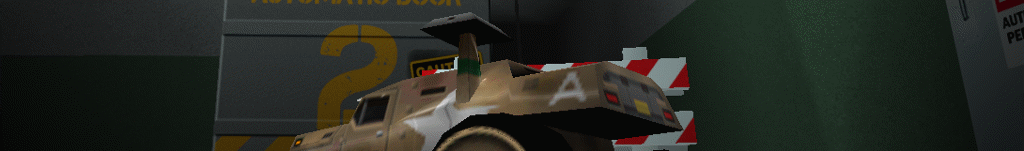Re-Volt I/O

# Car Spinner

Edit
Date: January 9, 2017
Authors: Gel38
Categories: Parameters
Tags: car_making``````;====================
; Car spinner details
;====================

SPINNER { ; Start spinner
ModelNum -1
Offset 0.000000 0.000000 0.000000
Axis 0.000000 1.000000 0.000000
AngVel 0.000000
} ; End Spinner``````

### 1. ModelNum

This corresponds with the "Model Filenames" section. This setting uses the numbers -1 to 18 and determines which model is used. If no models are used, this number should be set to -1, otherwise from 0 to 18. In this case you want the number corresponding with the spinner prm.

### 2. Offset

These numbers determine the placement of your 3d spinner model. It uses an XYZ coordinate system.

X-0, Z-0, Y-0

X = width of car (This is the 1st number) Y = length of car (The 3rd number) Z = height of car(2nd number)

Positive numbers on the X axis place the model to the Right, whereas, Negative numbers place it to the Left.

Positive numbers on the Z axis will move the model down toward the ground, whereas, Negative numbers will bring it up.

Positive numbers on the Y axis move the model forward, whereas, Negative numbers it to the rear

### 3. Axis

These numbers range from 0 to 1. This is the axis (X,Y, or Z) on which the spinner model should rotate. It is possible to combine 2 or 3 axis to achieve unique angles.

Numbers less than 1 or greater than 1 will cause the model to be scaled to that size when turning. An input of 2 will cause the spinner to not only rotate, but at full rotation, become twice it's original size. An input of 0.5 will cause the model, at full rotation, to be scaled to half of it's original size.

### 4. AngVel

This is the velocity (or speed) of the spinner rotation. A value of 1 means that the spinner will spin once in 1 second. A value of 2 means 2 rotations in one second, and so forth. A negative value will make the spinner spin in the opposite direction.